Abstract: Being one of the most commonly-used tools in describing the dynamic system, the delay differential equation contains not only the current value of the signal but also the previous one. However, it cannot be directly solved by writing anonymous functions with Matlab if the delay differential equation is only one part of the complex system, and its input signal comes from the previous module; therefore, the simulation strategy on the basis of the box diagram is the best way to solve such problems. Based on the box diagram, Matlab Simulink is the ideal tool, which helps build the delay differential equation model. By this way, we can solve the numerical solution of this equation.

1. 引言

2. 典型延迟微分方程的数值求解

${x}^{\prime }\left(t\right)=f\left[t,x\left(t\right),x\left(t-{\xi }_{1}\right),x\left(t-{\xi }_{2}\right),\cdots ,x\left(t-{\xi }_{n}\right)\right],$

$\left\{\begin{array}{l}{{x}^{\prime }}_{3}\left(t\right)=4{x}_{1}\left(t\right)-2{x}_{2}\left(t\right)-3{x}_{3}\left(t\right)\\ {{x}^{\prime }}_{2}\left(t\right)={x}_{3}\left(t\right)\\ {{x}^{\prime }}_{1}\left(t\right)=0.2{x}_{1}^{3}\left(t-0.5\right)-{x}_{1}\left(t-0.5\right)-3{x}_{1}\left(t\right)-{x}_{2}\left(t-1\right)-1\end{array}$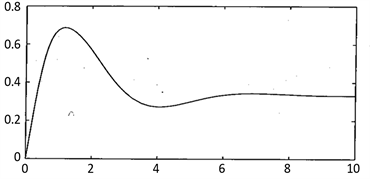(a)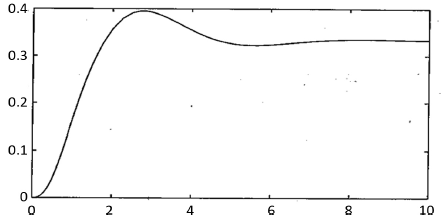(b)

Figure 1. (a) The numerical solution of the delay differential equation (The initial condition is zero); (b) The numerical solution of the delay differential equation (The initial condition is non-zero)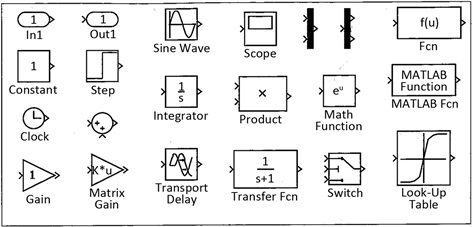Figure 2. Commonly-used custom module groups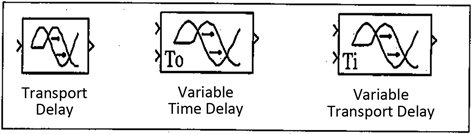Figure 3. The Various delay modules provided by continuous function groups in Simulink

$\left\{\begin{array}{l}4x\left(t\right)=2y\left(t\right)+3{y}^{\prime }\left(t\right)+{y}^{″}\left(t\right)\\ {x}^{\prime }\left(t\right)=0.2{x}^{3}\left(t-0.5\right)-x\left(t-0.5\right)-3x\left(t\right)-y\left(t-1\right)-1\end{array}$

$3x\left(t\right)+{x}^{\prime }\left(t\right)=0.2{x}^{3}\left(t-0.5\right)-x\left(t-0.5\right)-y\left(t-1\right)-1$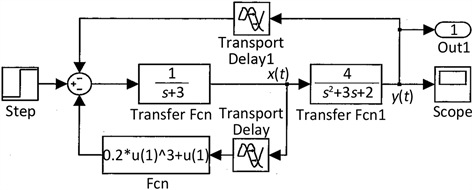Figure 4. Simulink Modeling of the delay differential equation

$>>\left[t,x\right]=sim\left(\text{'}c7mdde2\text{'},\left[0,10\right]\right)$

$plot\left(t,x\right)$ ； %绘图显示仿真结果

${x}^{\prime }\left(t\right)={M}_{1}\cdot x\cdot \left(t-0.15\right)+{M}_{2}\cdot {x}^{\prime }\cdot \left(t-0.5\right)+K\cdot u\left(t\right),$

${M}_{1}=\left[\begin{array}{ccc}-13& 3& -3\\ 106& -116& 62\\ 207& -207& 113\end{array}\right],\text{\hspace{0.17em}}{M}_{2}=\left[\begin{array}{ccc}0.02& 0& 0\\ 0& 0.03& 0\\ 0& 0& 0.04\end{array}\right],\text{\hspace{0.17em}}K=\left[\begin{array}{c}0\\ 1\\ 2\end{array}\right],$

$>>M1=\left[-13,3,-3;106,-116,62;207,-207,113\right];$

$M2=diag\left[0.02,0.03,0.04\right];$

$K=\left[0,1,2\right];$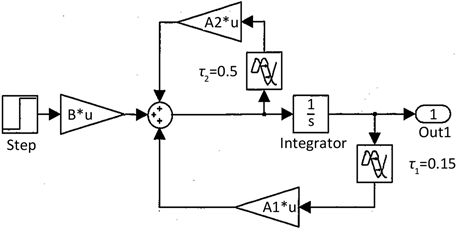Figure 5. Simulink Modeling with the delay differential equation

$>>\left[t,x\right]=sim\left(\text{'}c7mdde3\text{'},\left[0,8\right]\right)$

$plot\left(t,x\right)$ ； %将仿真结果绘图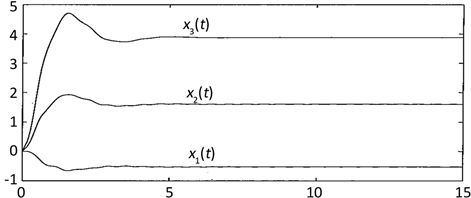Figure 6. The Simulated experimental result of the delay differential equation

$\left\{\begin{array}{l}{{x}^{\prime }}_{1}\left(t\right)=-2{x}_{2}\left(t\right)-3{x}_{1}\left(t-0.2|\mathrm{sin}t|\right)\\ {{x}^{\prime }}_{2}\left(t\right)=-0.05{x}_{1}\left(t\right){x}_{3}\left(t\right)-2{x}_{2}\left(t-0.8\right)+2\\ {{x}^{\prime }}_{3}\left(t\right)=0.3{x}_{1}\left(t\right){x}_{2}\left(t\right){x}_{3}\left(t\right)+\mathrm{cos}\left[{x}_{1}\left(t\right){x}_{2}\left(t\right)\right]+2\mathrm{sin}\left(0.1{t}^{2}\right)\end{array}$

$x\left(t\right)$${x}_{1}\left(t-0.2|\mathrm{sin}t|\right)$${x}_{2}\left(t-0.8\right)$ ，t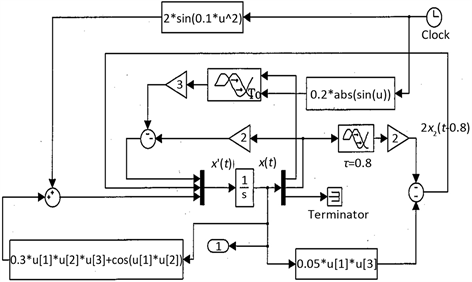Figure 7. The Simulink model of the variable time delay differential equation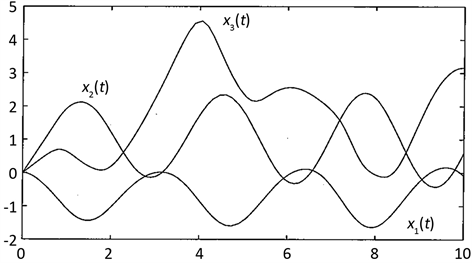Figure 8. The simulated experimental numerical solution of the variable time delay differential equation

 薛定宇, 陈阳泉. 基于MATLAB/Simulink的系统仿真技术与应用[M]. 第2版. 北京: 清华大学出版社, 2011: 7-12.

 王旭, 王宏, 王文辉. 人工神经元网络原理与应用[M]. 沈阳: 东北大学出版社, 2000: 179-185.

 薛定宇. 分数阶微积分学与分数阶控制[M]. 北京: 科学出版社, 2018: 33-39.

 邵军力, 张景, 魏长华. 人工智能基础[M]. 北京: 电子工业出版社, 2000: 217-222.

 薛定宇. 高等应用数学问题的MATLAB求解[M]. 第四版. 北京: 清华大学出版社, 2018: 317-326.

Top